# 硬核！手写一个优先队列

## 前言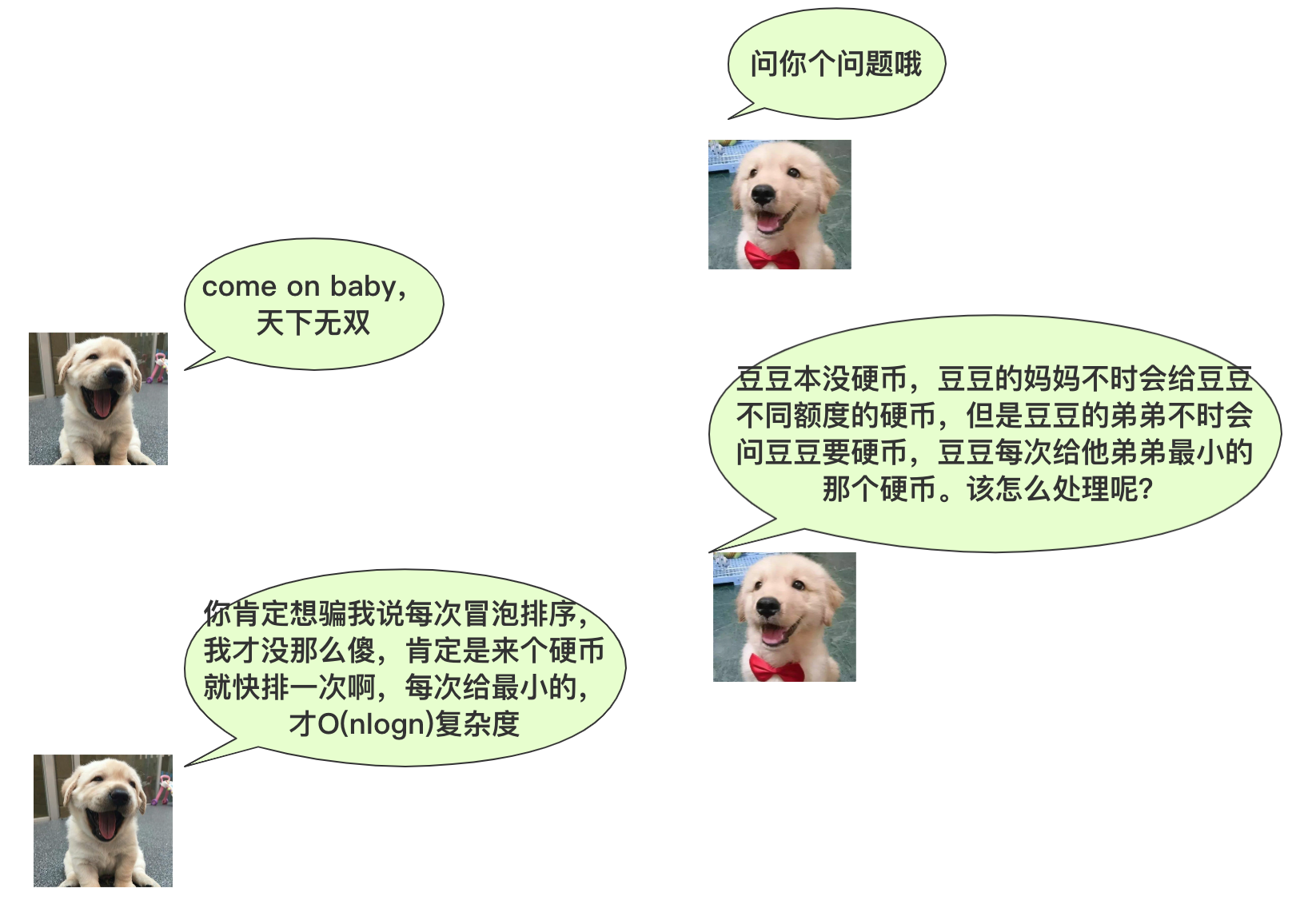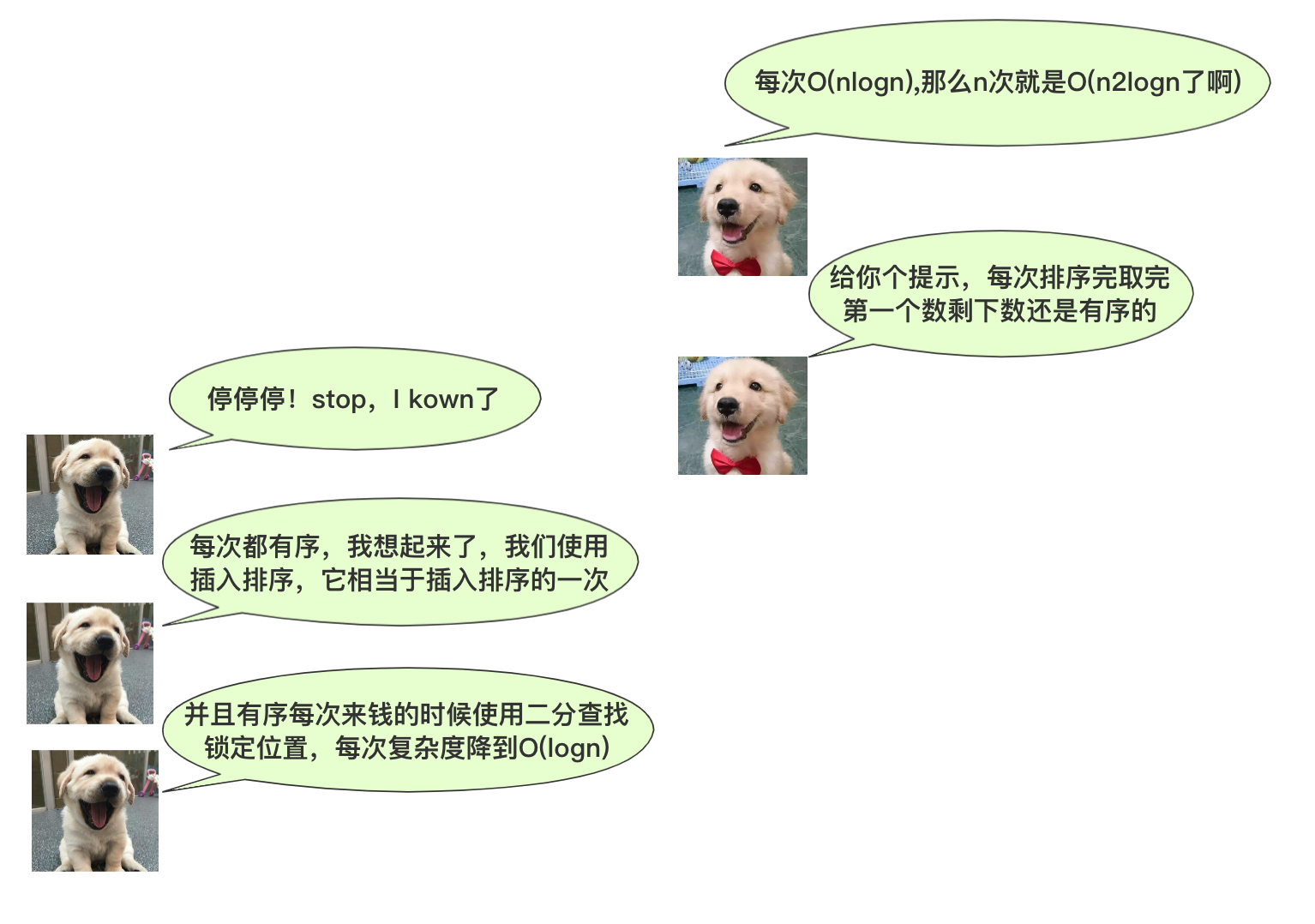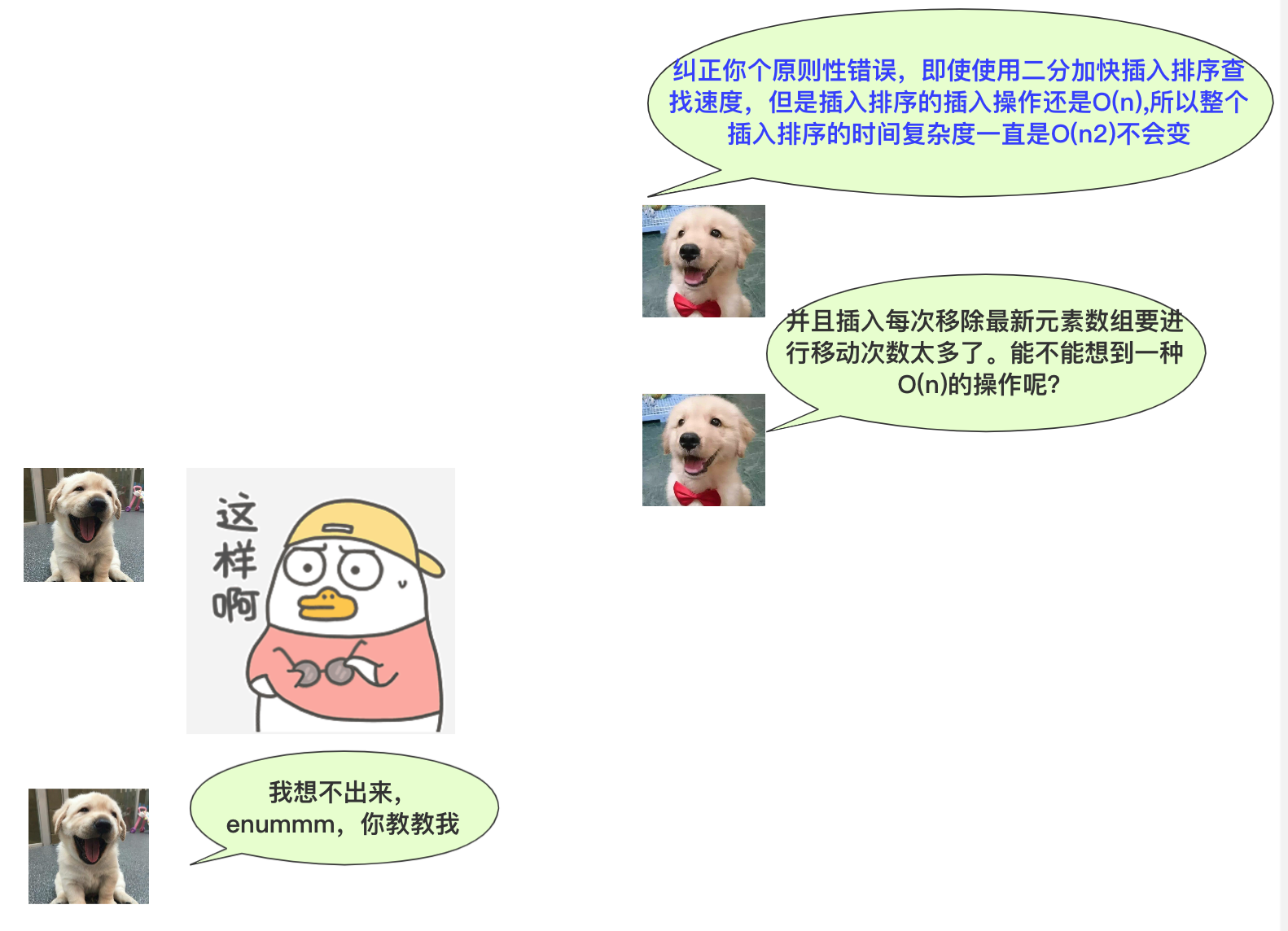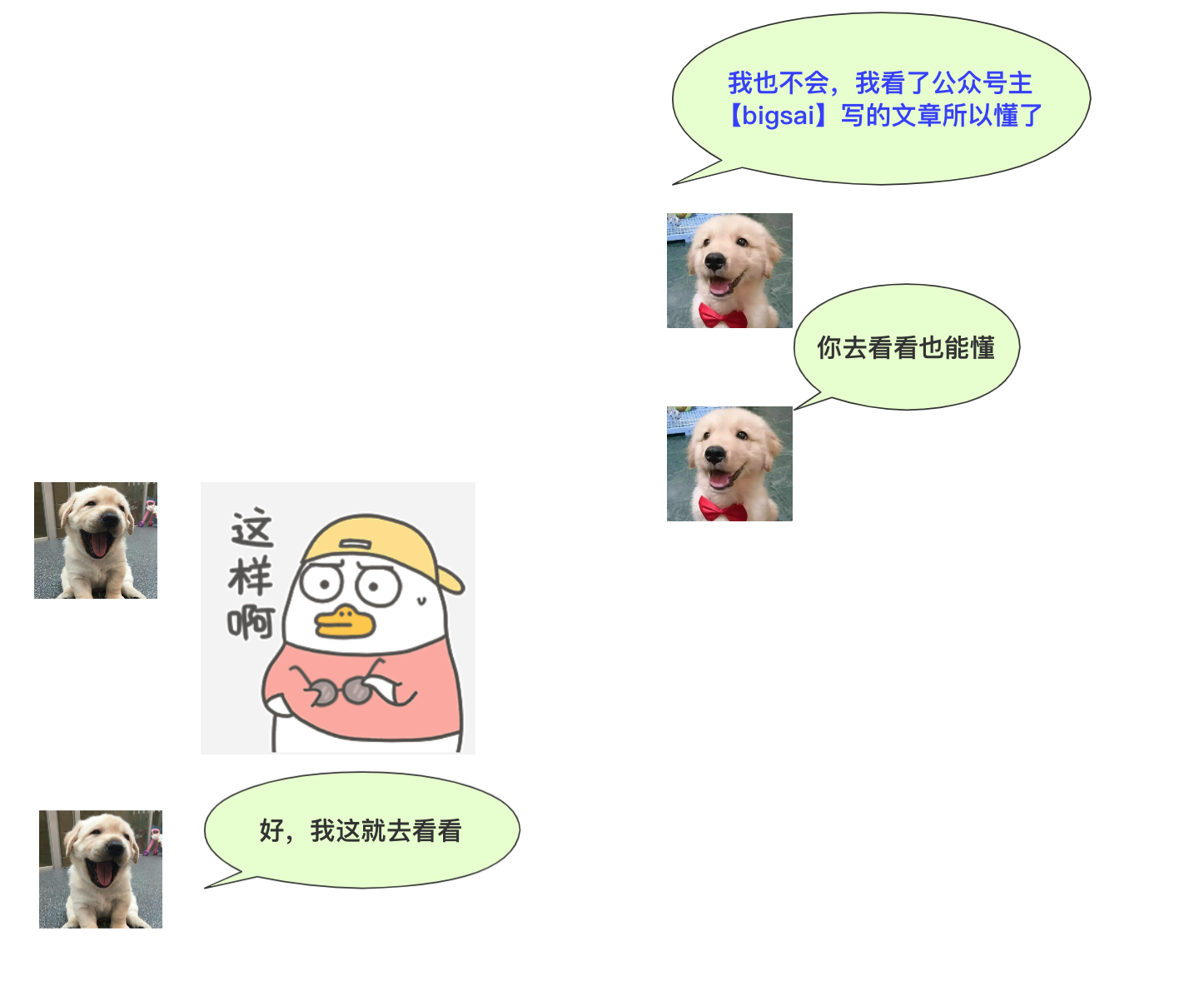## 堆

• 堆总是一棵完全二叉树
• 每个节点总是大于(或小于)它的孩子节点。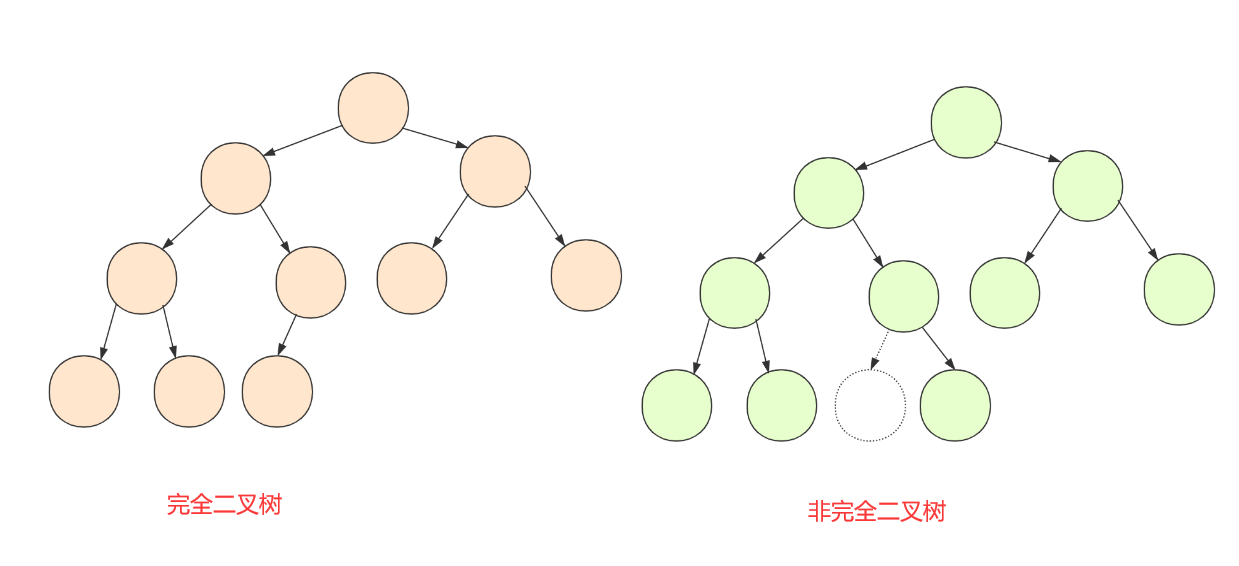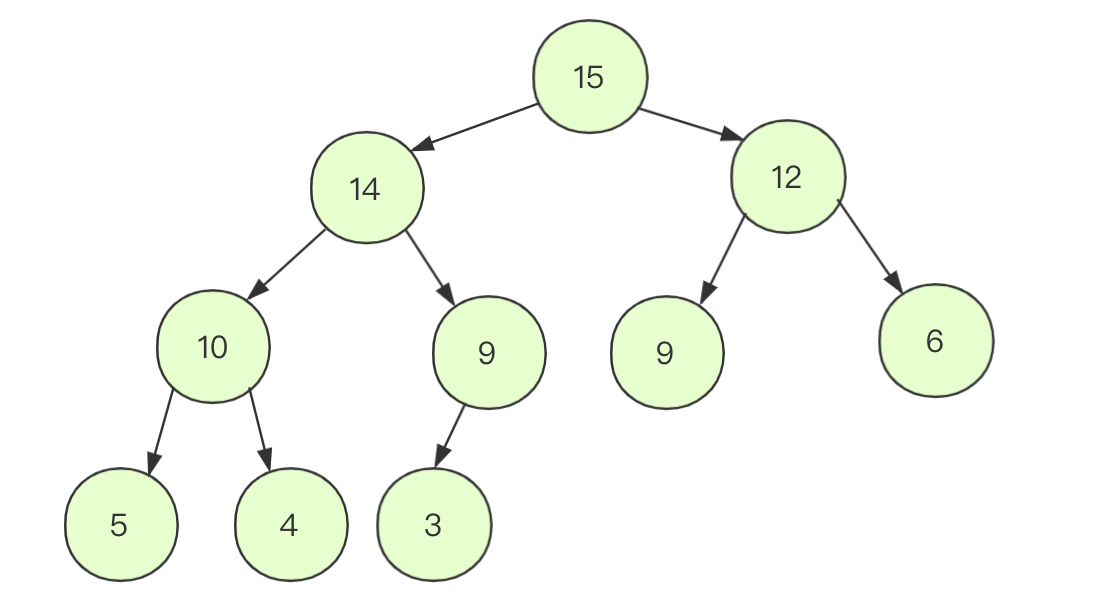## 优先队列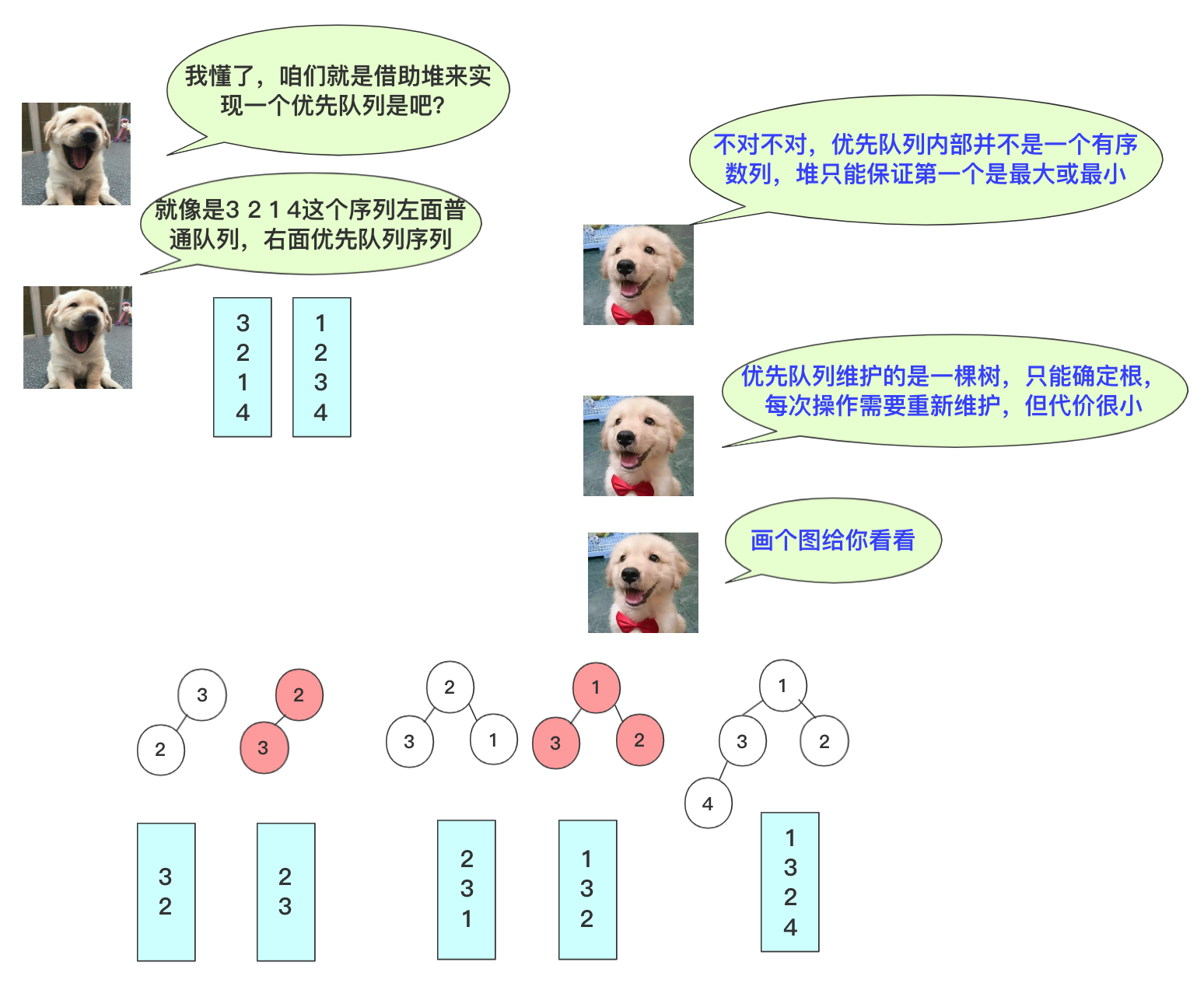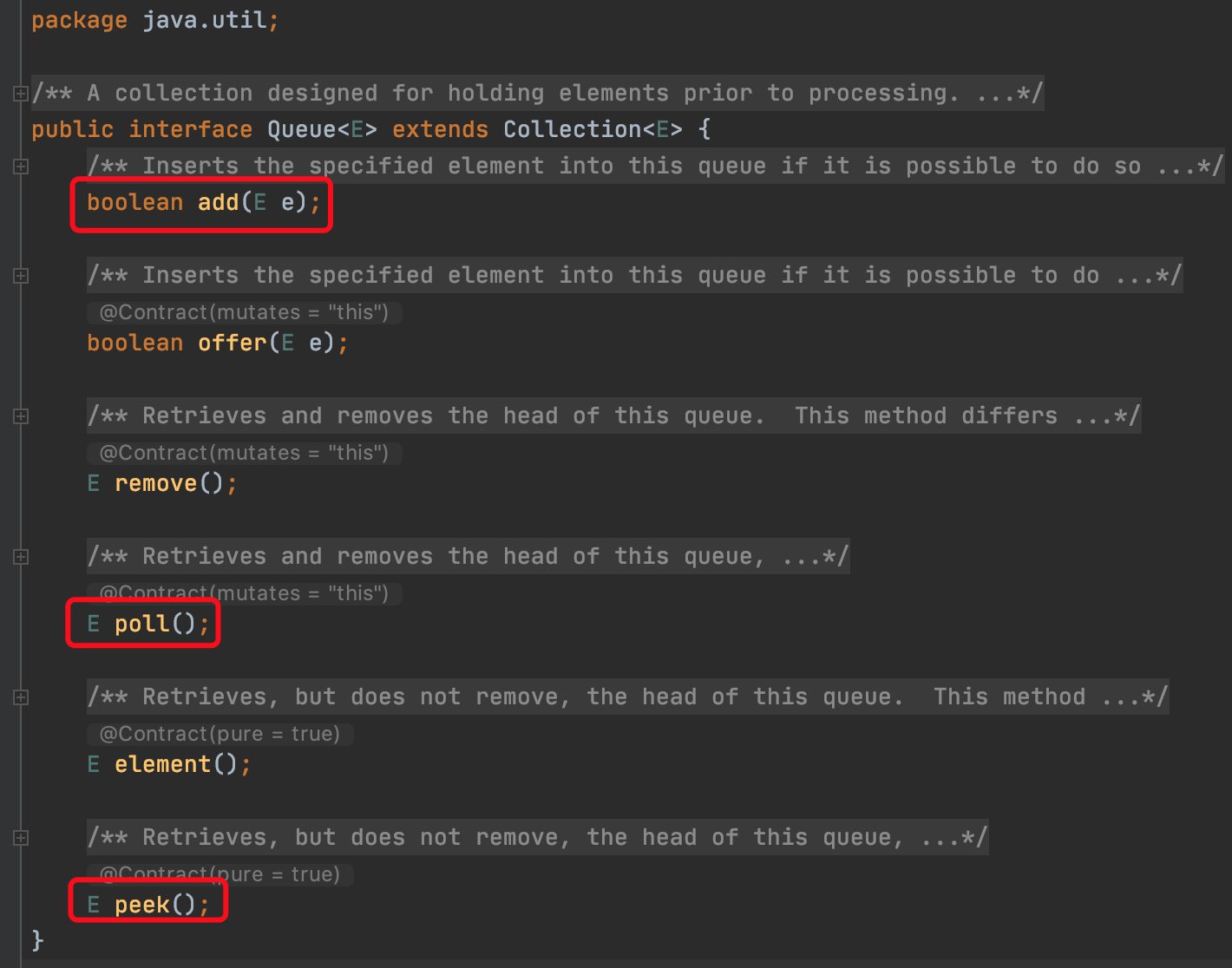• 刚开始是一个杂乱无章的序列，所以需要将杂乱的序列（树）通过一个方法变成一个合法的堆。
• 转成一个堆之后需要删除n次每次删除完都要重新调整这个堆。没有插入操作

• 队列（堆）刚开始的内容为空，每次增加一个元素时需要即使调整堆。每次删除也要及时调整堆，增加和删除每次都只是一个元素。

• 正常处理完的优先队列内的数据满足一个堆的结构，所以就是插入在堆中。
• 堆是一棵完全二叉树，所以在插入初始，插入到最后一个位置不影响其他结构。
• 节点和父节点比较大小(父节点索引为其二分之一)。如果该节点比父节点更小，则交换数据，一直到不能交换为止，这个过程不用担心不合法，因为父节点更小的话更满足比孩子节点更小。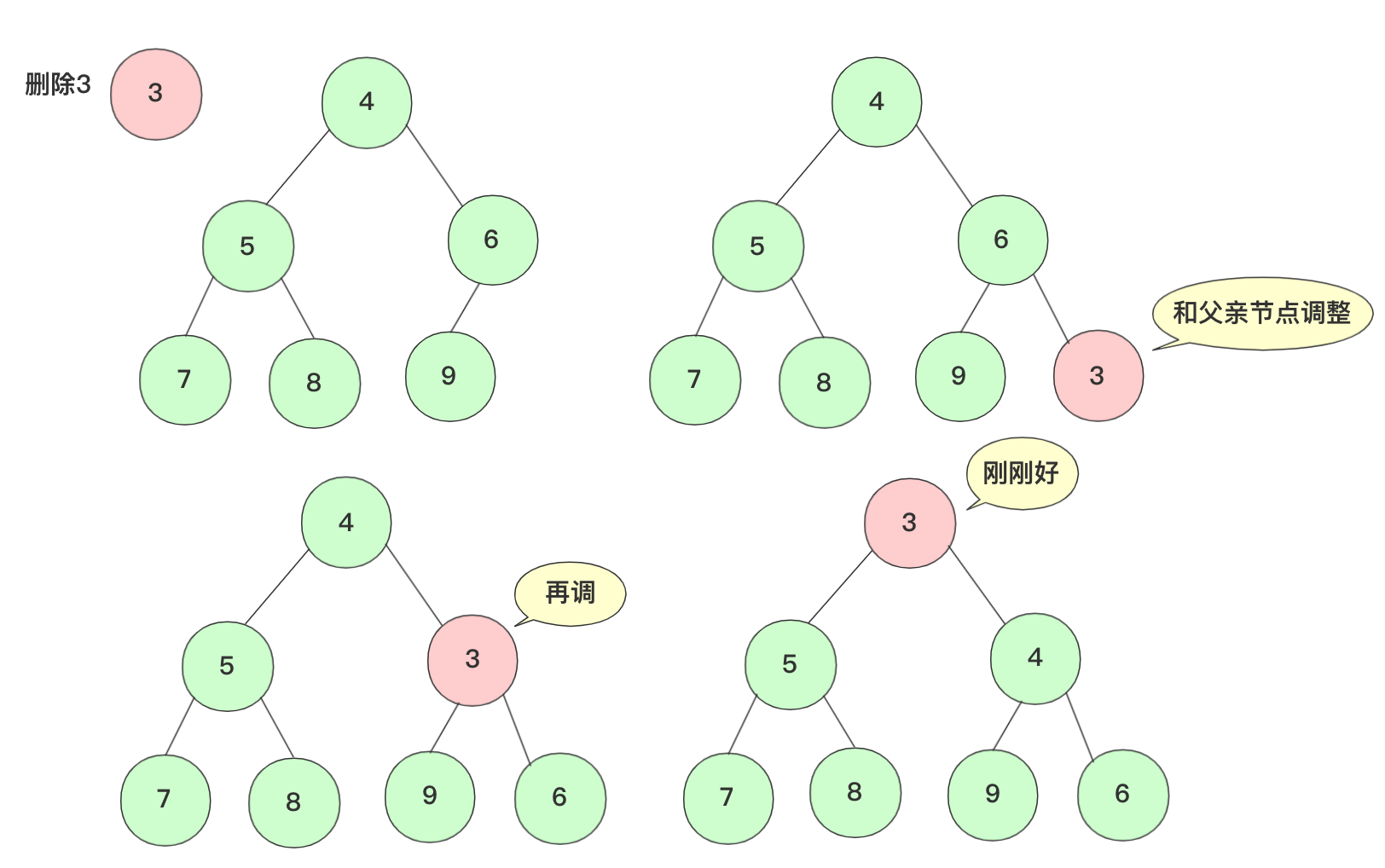• pop删除操作取优先队列内最小的元素，而这个元素肯定就是堆顶元素了，取完之后，这个堆的其他部分还是满足堆的结构但是缺少堆顶。
• 为了不影响整个结构，我们将末尾的那个元素移到堆顶，此时堆需要调整使其满足堆的性质条件。
• 交换的这个节点和左右孩子进行比较，如果需要交换则交换，交换后再次考虑交换子节点是否需要交换，一直到不交换为止。最坏情况是交换到根节点，这个复杂度每次为O(logn).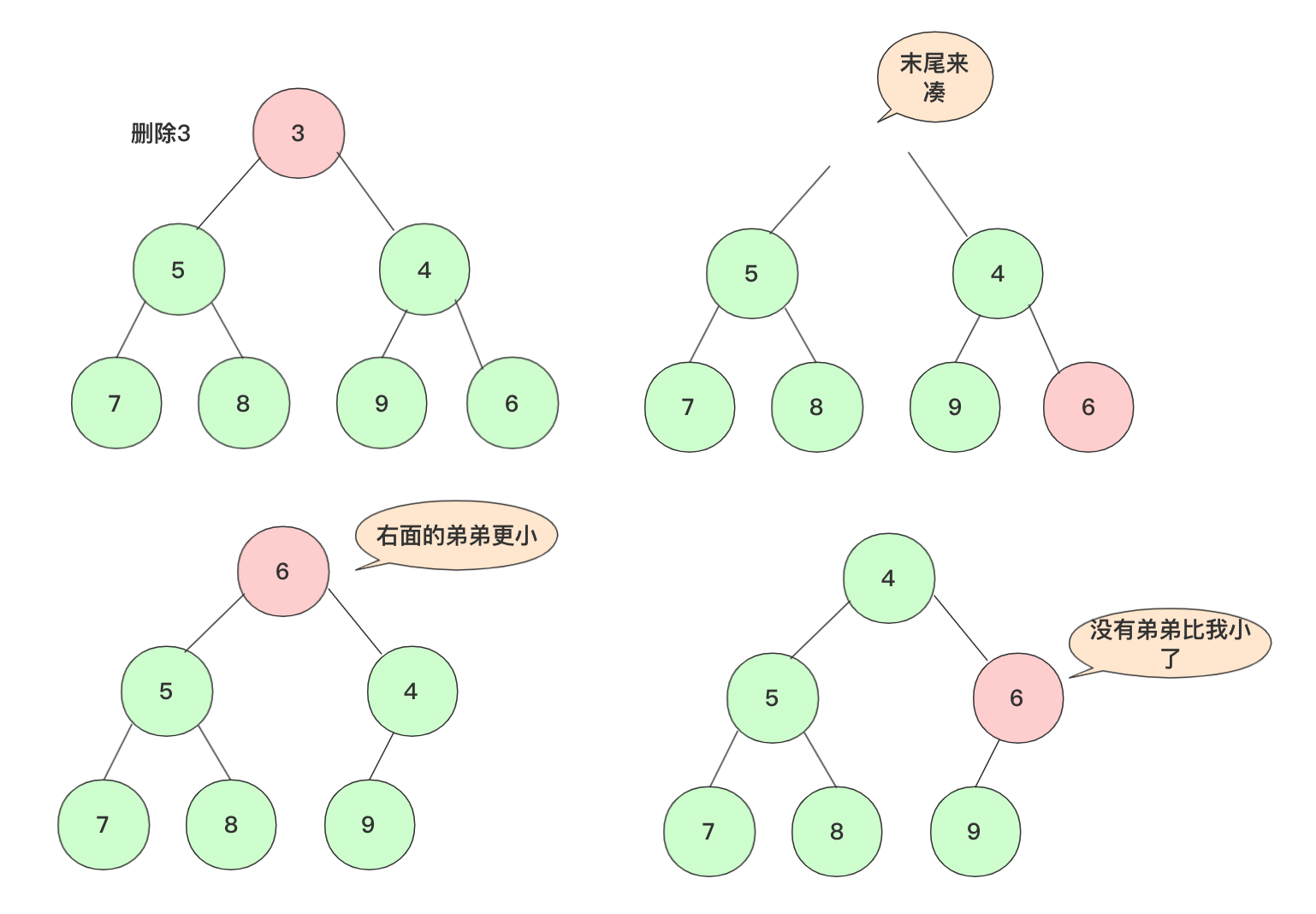## 代码实现

import java.util.Arrays;

public class priQueue {

private  int size;//优先队列的大小
private  int capacity;//数组的容量
private  int value[];//储存的值

public priQueue() {
this.capacity = 10;
this.value = new int[capacity];
this.size=0;
}
public priQueue(int capacity) {
this.capacity = capacity;
this.value = new int[capacity];
this.size=0;
}

/**
* 插入元素
* @param number
*/
if(size==capacity)//扩容
{
capacity*=1.5;
value= Arrays.copyOf(value,capacity);
}
value[size++]=number;//先加到末尾
int index=size-1;
while (index>=1) {//进行交换
if(value[index]<value[index/2]) {
swap(index,index/2,value);
index=index/2;
}
else//不需要交换即停止
break;
}
}
public int peek() {
return  value;
}

/**
* 抛出队头
* @return
*/
public int pop() {
int val=value;//呆返回数据额
value=value[--size];//将最后一个元素赋值在堆头
int index=0,leftChild=0,rightChild=0;
while (true)
{
leftChild=index*2+1;
rightChild=index*2+2;
if(leftChild>=size)//左孩子必须满足在条件内
break;
else if(rightChild<size&&value[rightChild]<value[index]&&value[rightChild]<value[leftChild])
{//右孩子更小
swap(index,rightChild,value);
index=rightChild;
}
else if(value[leftChild]<value[index])
{//左孩子更小
swap(index,leftChild,value);
index=leftChild;
}
else //不需要 它自己最小
break;
}
return  val;
}
//交换两个元素
public  void swap(int i,int j,int arr[]) {
int team=arr[i];
arr[i]=arr[j];
arr[j]=team;
}

public int size() {
return  size;
}
}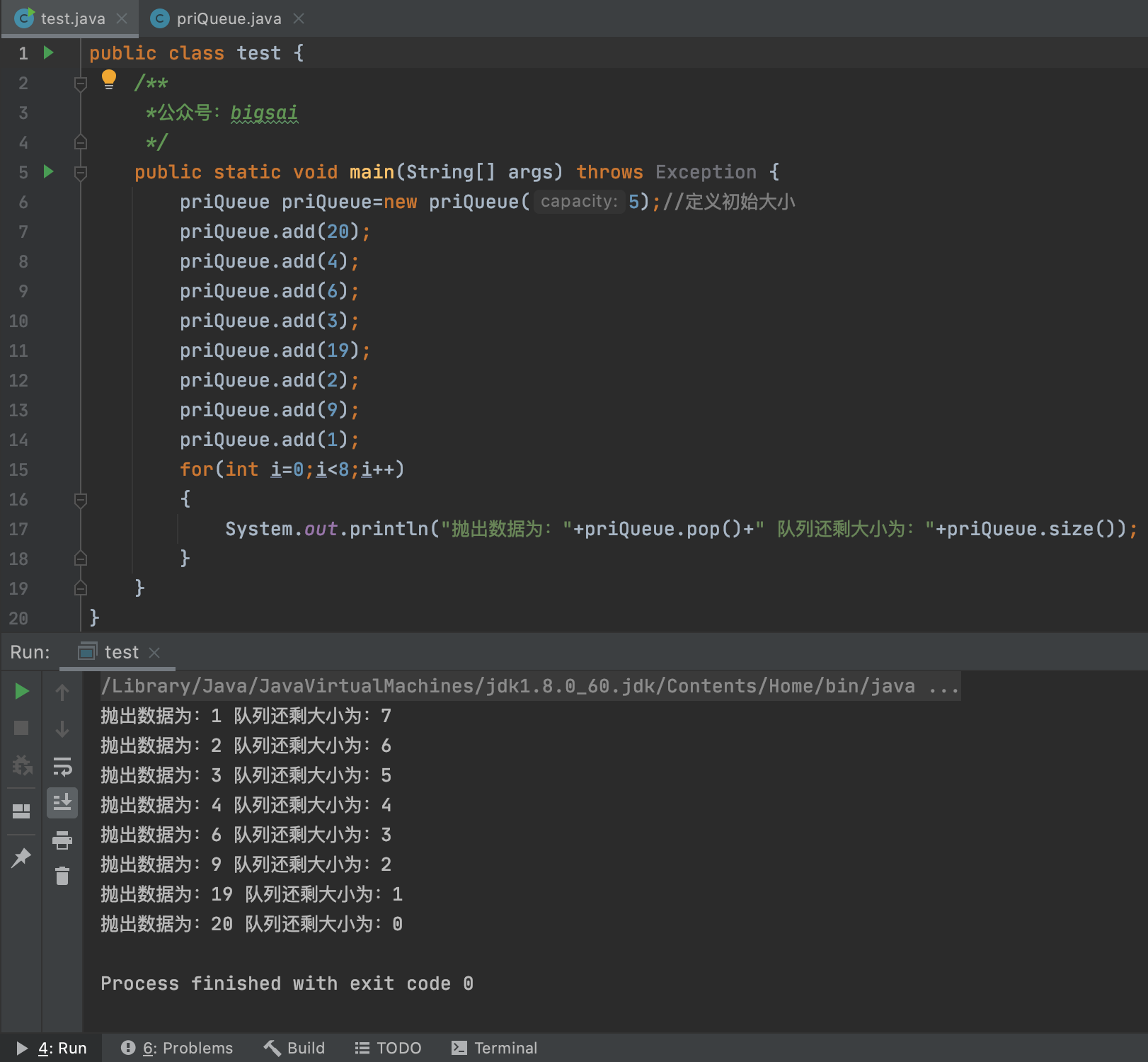## 结语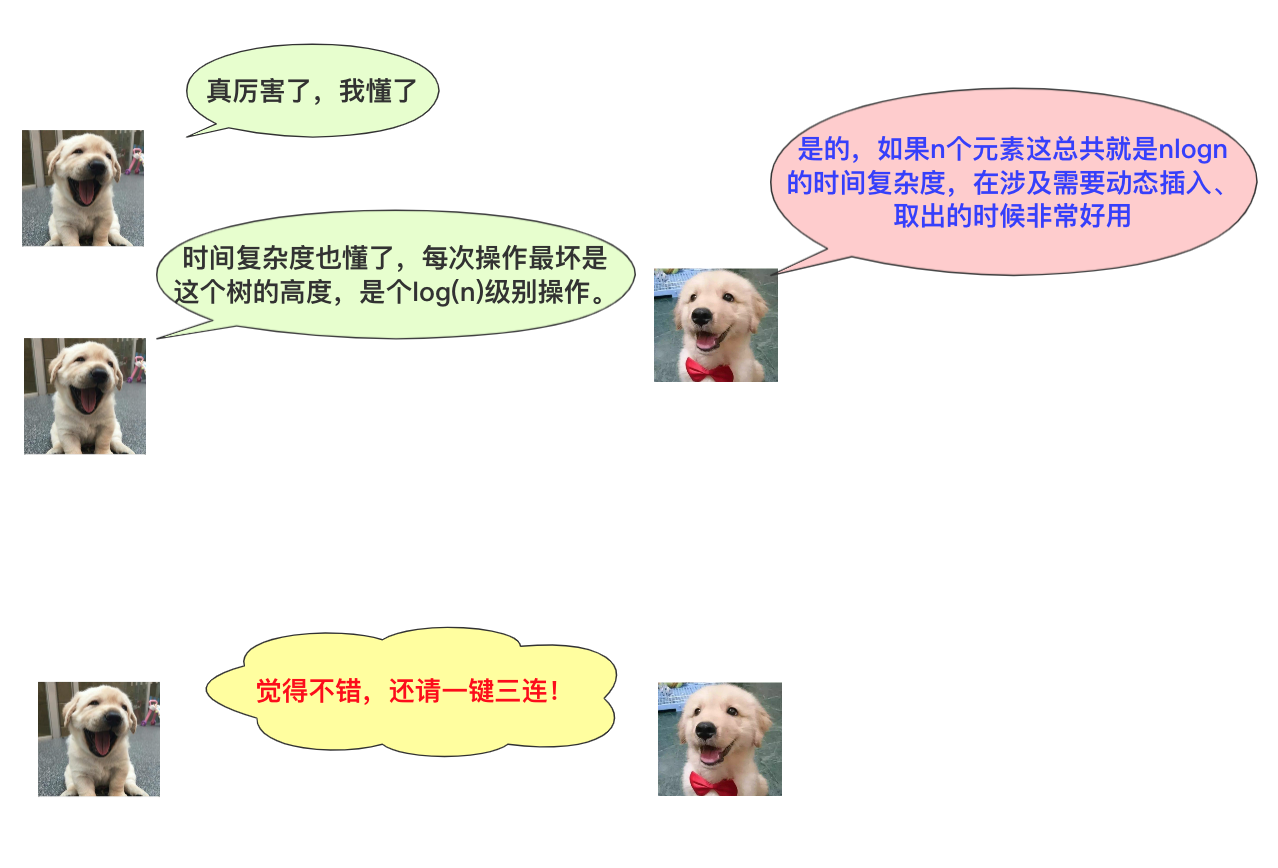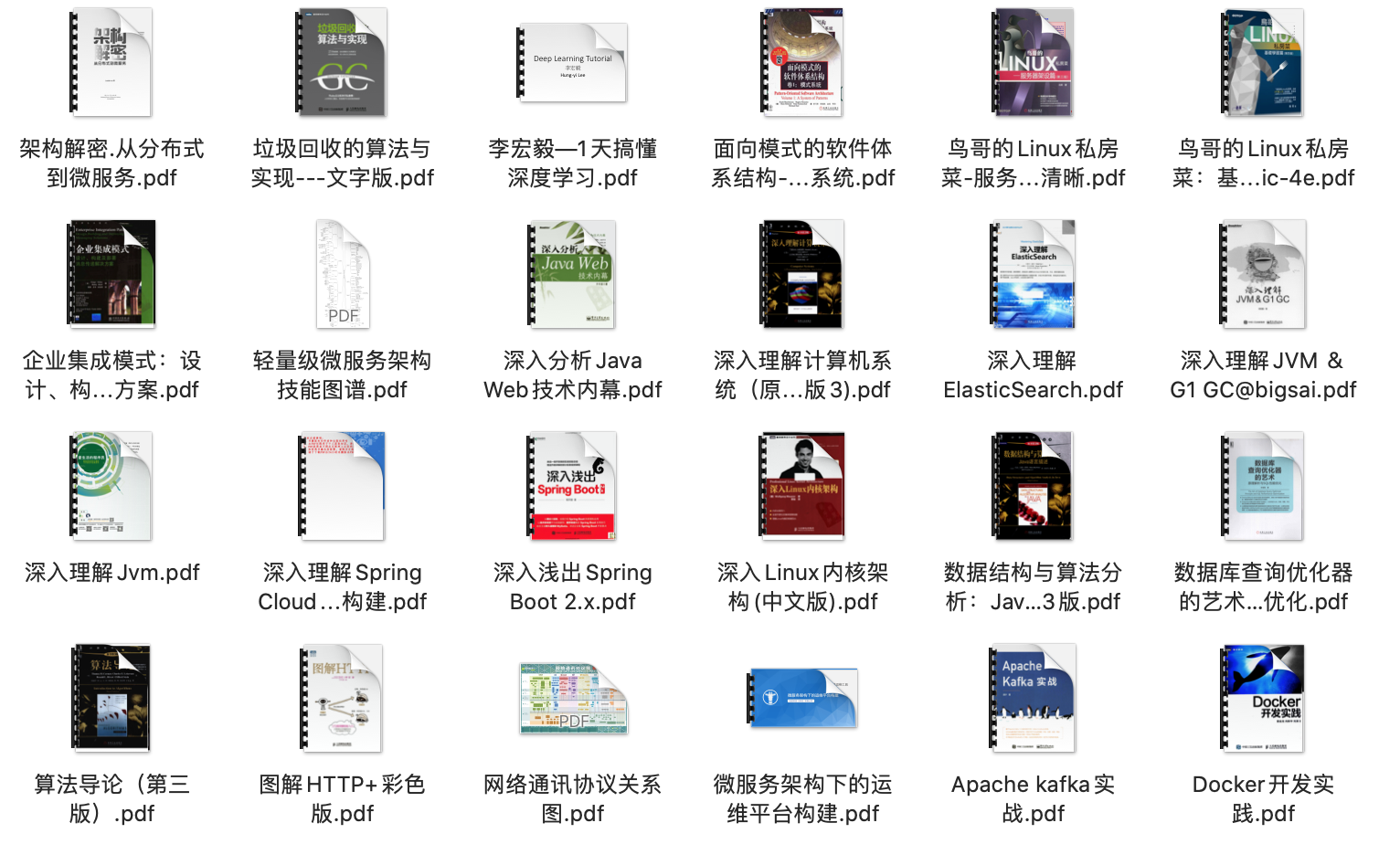Big sai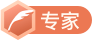CSDN认证博客专家 数据结构与算法 爬虫 Java

07-24318005-284451
09-191754
09-04807
04-23174
05-12361
02-1965
04-18453
11-2514点击重新获取扫码支付余额充值